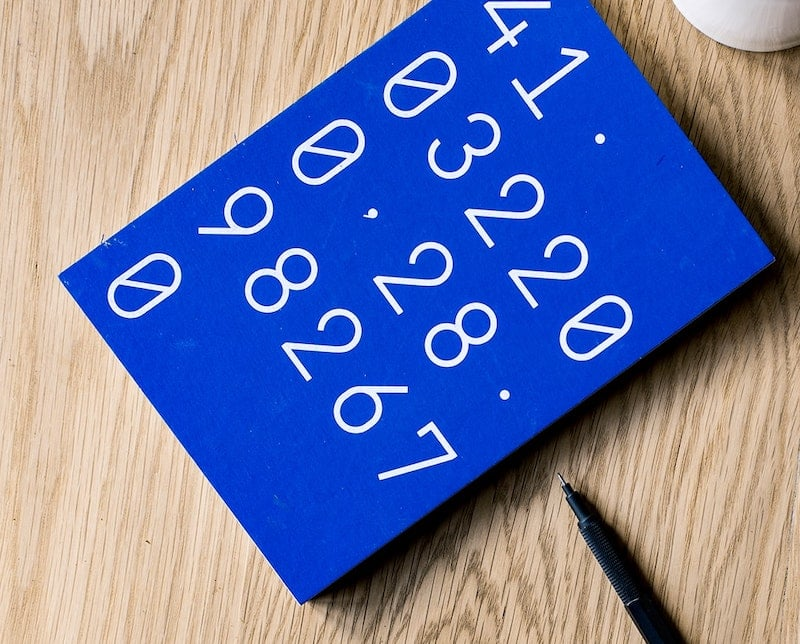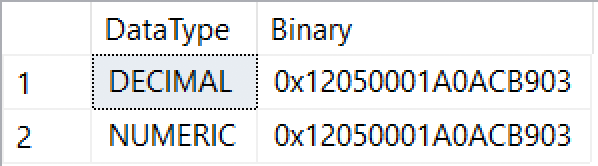Home » How SQL Server stores data types: integers and decimals

# How SQL Server stores data types: integers and decimalsThis week we’re going to look at how numbers are stored. This post will cover integers (`TINYINT`, `SMALLINT`, `INT`, `BIGINT`), and decimals (`DECIMAL`, which is the same as `NUMERIC`).

If you’d like to read the previous posts in this series of how SQL Server stores data types, here’s what we’ve covered:

As a general reminder, there are eight bits in a byte.

### Integers

As we know from before, integers are whole numbers, or numbers with no fractions (i.e. no decimal places). This is going to be in the test later, so pay attention. In other words, the numbers 0 through 9 are integers, but a floating point or decimal / numeric value is not an integer. As soon as you add decimal places, it stops being an integer even if the fraction equates to zero.

Inside the storage engine, integers are mostly signed values (they can have negative values), and each integer data type has a fixed size. The exception is `TINYINT` which only has positive values. Like many other data types, integer types are stored byte-reversed (known as little-endian).

 Type Size Decimal range Binary range `TINYINT` 1 byte 0 to 255 0 to 2^8 – 1 `SMALLINT` 2 bytes -32,768 to 32,767 -2^15 to 2^15 -1 `INT` 4 bytes -2,147,483,648 to 2,147,483,647 -2^31 to 2^31 – 1 `BIGINT` 8 bytes -9,223,372,036,854,775,808 to 9,223,372,036,854,775,807 -2^63 to 2^63 – 1

Integers are accurately represented in binary. For instance, the number 625 is represented in binary as `00000010 01110001`, or `0x0271` in hexadecimal. We use hex as a representation of binary to save space when displaying binary values, but it’s purely at the presentation layer. Every byte is always eight bits wide. A byte is represented by a 2-digit value in hexadecimal. `271` (the hex equivalent of 625) is more than one byte, so we put a leading zero in front of it to round it up to the nearest byte, and use those two bytes to store the value.

625 is ten bits wide, so it is too large for `TINYINT`‘s boundary of eight bits in the below example. For the rest though, it will look pretty much the same, just with extra zeroes padded to the right. Let’s take a look:

 Type Value Hex `TINYINT` 255 `0xFF` `SMALLINT` 625 `0x7102` `INT` 625 `0x71020000` `BIGINT` 625 `0x7102000000000000`

Negative values use two’s complement, which we covered briefly last time. It uses the high end of the byte range to store those negative values, starting at -1.

The smallest negative value (-1) has the largest possible hex value (`0xFF` for each byte), and then counts backwards from there to the lowest possible value. So for the `SMALLINT` data type, -1 is `0xFFFF`, and -2 is `0xFFFE` (which is stored as `0xFEFF` byte-reversed). The binary values converge at the maximum positive value, where `SMALLINT`‘s maximum is 32,767 (`0x7FFF`, but stored as `0xFF7F`) and -32,768 is `0x8000`, but stored as `0x0080`.

Two’s complement is a common technique for storing signed integers to make the best use of the available bytes, and it is why understanding data types is extremely important when converting between signed and unsigned values, and casting between different data types. Endianness certainly complicates matters.

### Fixed-precision numerics

In SQL Server, the precision and scale of a `DECIMAL` (the same thing as a `NUMERIC`) data type matters a great deal. As far as SQL Server is concerned, `DECIMAL(19,4)` is a different data type to `DECIMAL(18,5)`, and is stored differently.

As we discussed in a previous post, the precision is the total length being stored including the decimal places, while the scale is the number of digits after the decimal point. So for example, `DECIMAL(19,4)` has a precision of 19 and a scale of 4, which means it can store up to 15 digits before the decimal point, followed by up to four digits after the decimal point. If a number with more decimal places is stored in that data type, it will be rounded up.

The precision affects the number of bytes required to store the value, ranging from 5 bytes (precision 1 – 9) to 17 bytes (precision 29 to 38). If 17 bytes sounds like a lot, that’s because SQL Server needs to maintain the accuracy for those high-precision values, especially when it comes to financial and statistical calculations.

If you need to know what value is being stored in a column, the scale is the incrementing value. For a decimal column with a precision of 19 and scale of 4, it will require nine bytes to store the amount. The bytes are stored in reverse (little-endian), and the value will increment in 0.0001 increments (the scale of 4), which looks as follows:

 Value Hex 0.0000 `0x010000000000000000` 0.0001 `0x010100000000000000` 0.0002 `0x010200000000000000` 0.0003 `0x010300000000000000` 625.0000 `0x01105E5F0000000000` 3000000000.0000 `0x0100E057EB481B0000` 3000000000.0001 `0x0101E057EB481B0000` –3000000000.0000 `0x0000E057EB481B0000` –3000000000.0001 `0x0001E057EB481B0000`

Notice that positive values have `0x01` in the first position, while negative values have `0x00` in the first byte position. Other than that, positive and negative values are identical. This is different to the use of two’s complement in other data types.

Let’s check out the 3 billion value, and remove the leading signed bit: `0x00E057EB481B0000`. To look at it in a hex calculator, we need to reverse it to `0x 1B 48 EB 57 E0 00`. Putting that into a hex calculator gives us `30,000,000,000,000`. Knowing that the scale is 4, we move the decimal point four spaces to the left, and we have our expected value of 3 billion.

#### The CAST AS VARBINARY problem

The data type on disk makes sense as a byte-reversed integer, because the table definition contains the column information in the metadata. However when we cast a `DECIMAL` as `VARBINARY`, things look very different. That’s because — like with the `TIME` and `DATETIME2` data types — the precision and scale needs to be encoded in the binary value to ensure the accuracy is retained.

The bottom line is that you cannot assume that the value you see in a `VARBINARY` cast is how that value is stored on disk.

That said, I keep saying that `DECIMAL` and `NUMERIC` are the same thing, so let’s prove it with some Transact-SQL that returns the values as binary data in hexadecimal format:

```SELECT 'DECIMAL' AS DataType, CAST(CAST(625 AS DECIMAL(18, 5)) AS VARBINARY(25)) AS Binary
UNION SELECT 'NUMERIC', CAST(CAST(625 AS NUMERIC(18, 5)) AS VARBINARY(25));```

And this is what you should see in the results:Same thing

Next time we’ll look at floating points (`FLOAT` and `REAL`), and money (`MONEY` and `SMALLMONEY`).

1.